Background
While the ordinary least squares estimator has many desirable properties, particularly unbiasedness, in certain settings, it can suffer from large variance. For example, if the data have more regressors than the length of the dataset (frequently referred to as the large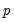, small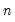problem), or if there are many correlated regressors, the least squares estimates are very sensitive to random errors and may have high variance. We can use regularization to reduce this variance by introducing bias, while lowering the total error, with an elastic net regression model.
Elastic net, Lasso, and ridge regression are all penalized regression methods that work by shrinking the magnitudes of the regressors in the model. The usual approach is to modify the standard cost function for linear regression with a penalty term.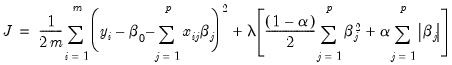(37.1)
Depending on the value of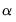in the penalty term, Equation (37.1) becomes a ridge regression model, a Lasso model, or an elastic net model. The magnitude of the penalty parameter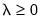controls the impact of the penalty. If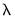is chosen to be a “large” value, the minimization of this cost function: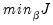(37.2)
will shrink or even set to zero the values of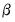. A model with smaller (or zero) coefficients is less complex and less prone to overfitting.
Note that the penalization does not include the constant (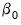) term. We only want to reduce the magnitudes of the regressors.
Ridge
The ridge estimator is the ordinary least squares estimator with an L2 penalty term attached: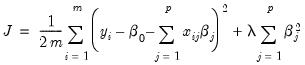(37.3)
The penalty shrinks the size of the coefficients but does not reduce them to zero. A biggerresults in more shrinkage when the cost function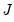is minimized.
While there are many ways of choosing the penalty parameter, for multipleEViews uses cross-validation. In short, this involves partitioning the data into training and test sets, looping over the list of parameters and estimating a set of coefficients with the training data. These coefficients are then used on the test data set to predict the dependent and calculate an error measure. The penalty parameter with the minimum error is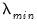. For cross-validation procedures with more than one test set we also calculate the standard error of the error measures across both the training and test sets (giving the largest penalty parameters within one and two standard errors ofas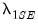and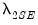). Additional information about the cross-validation options available in EViews will be discussed in the next section.
Analytic Solution
Solving the right-hand side of Equation (37.3) for the coefficients in the standard way yields: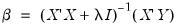(37.4)
Note that when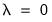, Equation (37.4) becomes the equation for the OLS coefficients, and when penalization parameter increases (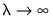), the coefficients are more heavily penalized (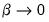). Conveniently, the addition of the positive constantto the diagonal makes the matrix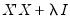nonsingular and invertible.
Bias is the difference between the expected value of the estimate and the actual value, and variance is the uncertainty in those estimates. The goal of regularization is to reduce model complexity to the extent that the model fits training data well but also generalizes well to test data. Low bias, more complex models tend to fit training data well, while low variance, less complex models tend to generalize better to test data. In OLS, for example, model complexity is related to the number of regressors. Reducing the number of regressors reduces the variance of the estimator at the cost of introducing bias. This is called the bias-variance tradeoff. For elastic net, ridge, and Lasso models, the equivalent reduction in complexity is achieved by a reduction in the magnitudes of the coefficients, not just in the elimination of coefficients themselves.
We can see this in more detail by calculating the expectation of the ridge coefficient with Equation (37.4):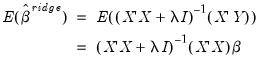(37.5)
Since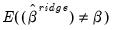, the ridge estimator is biased. We also see that as,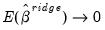, as expected.
While we will not derive them here, the bias and variance of the ridge estimator are given by: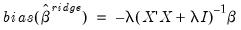(37.6)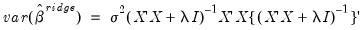(37.7)
Asincreases, the bias in Equation (37.6) increases, while the variance in Equation (37.7) (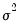is error variance from the residuals) decreases.
Lasso
The Lasso (Least Absolute Shrinkage and Selection Operator) estimator is the OLS estimator with an L1 penalty term: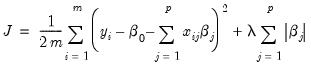(37.8)
Since the penalty term is a sum of absolute values the solution in nonlinear and there is no analytic solution. The Lasso equation must be solved numerically. Unlike in ridge regression, the coefficients can shrink to zero.
It is worth noting that if a group of regressors are correlated, Lasso will tend to favor one out of the group and shrink the others. Ridge regression will proportionally shrink the coefficients of the entire group.
Elastic Net
The elastic net model is a combination of the ridge and Lasso models. Repeating Equation (37.1):The regularization term is a combination of the L1 and L2 penalties, with the amount of mixing controlled by the parameter. When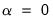, this becomes a ridge regression model. When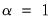, it becomes a Lasso model. The compromise between these two models works well for groups of correlated regressors, since the ridge term shrinks them proportionally while the Lasso term pushes them towards zero.